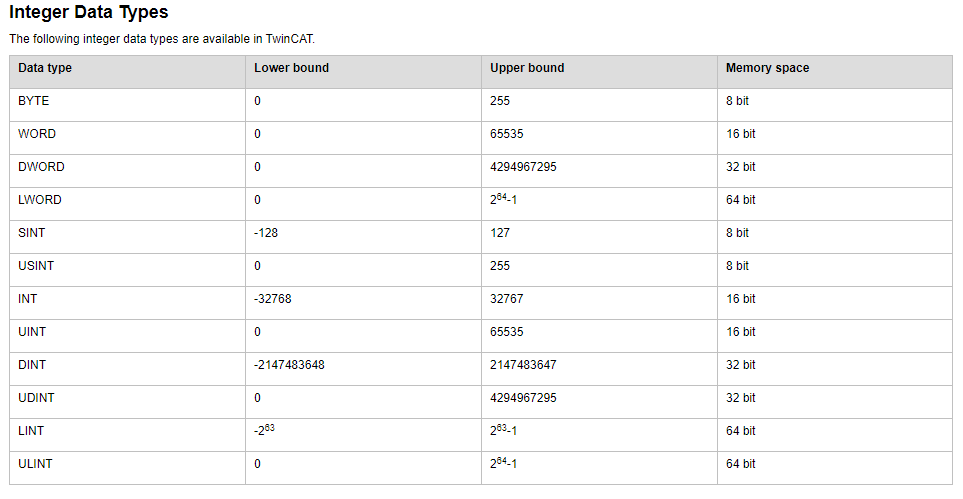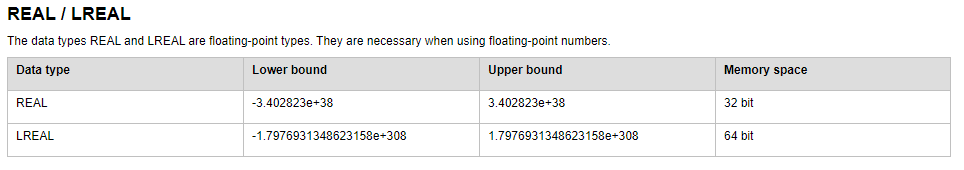PLC Data type:

Each program works with data. There are different types of data to match real world problem. For example a counter machine counts a number of objects (that can be 0 to n), on the other hand if we want to read pressure value from a pressure sensor that can be  for example  -20.5 bar to 200.01 bar. In this section we go through the different data types and it’s upper and lower range.

We shall consider all data types described in the IEC 61131-3 standard.

=> BOOL data type:

BOOL constants are the truth values TRUE (1) and FALSE (0).

=> INT data type:If we treat INT as 32 bit value, then we can have problem to write over ADS. For example, If we treat short to INT type which is 32 bit in windows then we shall have mismatch and causes problem in Visual Studio that may be difficult to remember.

`short iclwValue = 0; //if we write int icwValue = 0; will cause problem in ADStry{iclwValue = Int16.Parse(textBoxTargetCLW.Text);}catch (Exception){}          if (plc.IsPLCConnected) {object data = iclwValue;plc.WriteValue("MAIN.MachineType", data);`

=> REAL/LREAL Type:=>STRING Type:

A variable of data type STRING can accept any string. The size specification for the memory space allocation in the declaration refers to characters and is enclosed by round or square brackets. If no size is specified, TwinCAT assumes 80 characters by default.

As a rule, TwinCAT does not limit the string length, but the string function only processes lengths between 1 and 255! If a variable is initialized with a string that is too long for the data type of the variable, TwinCAT truncates the string from the right.

The memory space required for a STRING variable is always 1 byte per character + 1 additional byte, e.g. 81 bytes for a “STRING” declaration.

Example: String declaration with 35 characters

sVar : STRING(35) := ‘This is a String’;

=> WSTRING Type:

Unlike the data type STRING (ASCII), the data type WSTRING is interpreted in Unicode format. With WSTRING, a length of 10 means that the length of the WSTRING can occupy a maximum of 10 WORDs. In Unicode, however, for some characters several WORDs are required for encoding a character, so that the number of characters does not have to correspond to the length of the WSTRING (10 in this case).

Example:

wsVar : WSTRING := “This is a WString”;